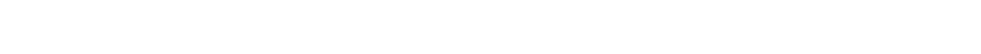###### Describe simple Calorimetry experiments for reactions such as combustion, displacement, dissolving and neutralisation

Calorimetry experiment: Used to measure heat transfer

#### Calorimetry Experiment for CombustionThe Calorimetry experiment for combustion

Method:

• Using a measuring cylinder, put 100 cm3 of water into a copper can
• Measure and record the initial temperature of the water
• Fill the spirit burner with test substance and measure and record its mass
• Place the burner under the copper can and light the wick
• Stir the water constantly with the thermometer and continue heating until the temperature rises by about 20 – 30°c and blow out the flame
• Measure and record the highest temperature of the water
• Measure and record the final mass of burner and remaining alcohol

Calculation:

Rise in temperature of water    =    final temperature – initial temperature

Mass of alcohol burnt     =    initial mass – final mass

Enthalpy change:Q – energy transferred to water

m – mass of water heated

c –  the specific heat capacity – is the amount of heat needed to raise the temperature of 1 gram of a substance by 1 oC.

For water, the value is 4.18 J g-1C-1 (joules per gram per degree Celsius).

∆T – change in temperature

When found Q you have calculated amount of heat released when you have burnt the mass of alcohol in the experiment.

You can work out:

Amount of heat released from 1g of substance = Q/mass of substance burnt.

Amount of heat released from 1 mole of substance = Q/mass of substance burnt x molecular weight of substance

#### Calorimetry Experiment for Displacement, Dissolving and NeutralisationThe Calorimetry experiment for displacement, dissolving and neutralisation

Method:

• Using a measuring cylinder, place 25 cm3 of solution 1 into a polystyrene cup
• Measure and record the temperature of solution 1
• Add a measured amount of reactant (solid for dissolving, solution 2 for displacement and Neutralisation) into the Polystyrene cup and stir the mixture
• Measure and record the highest temperature reached by the mixture

Calculation:

Rise in temperature    =    final temperature – initial temperature

Mass of solution  =  solution 1 + solution 2  or solution 1 if solid is dissolved

Enthalpy change:*If water is not used, this is replaced by the mass or volume of other solutions

Example calculations for the above experiment using sample data:

• Temperature of sulphuric acid: 15ºC
• Mass of calcium powder: 5g
• Mass of mixture: 32g
• Highest temperature of mixture: 23ºC
• Change in temperature: 23 – 15 = 8ºC
• Specific heat capacity of H2SO4: 1.34 J g-1 C-1
• Equation: Q = m x c x ΔT
• Q = 32g x 1.34J g-1 C-1 x 8ºC
• Q = 343.04 J

## Aiming for a Level 9?

See if you’ve got what it takes. Test yourself with our topic questions.

### Author: Jamie

Jamie got a First class degree in Chemistry from Oxford University before going on to teach chemistry full time as a professional tutor. He’s put together these handy revision notes to match the Edexcel IGCSE Chemistry specification so you can learn exactly what you need to know for your exams.